# 关于黄疸光疗仪光源光谱的研究Study on Light Source Spectrum of Jaundice Phototherapy Apparatus

• 全文下载: PDF(1154KB)    PP.201-209   DOI: 10.12677/OE.2018.84026
• 下载量: 184  浏览量: 502   科研立项经费支持

We establish an optical model of human skin to more effectively employ light energy when using phototherapy to treat neonatal jaundice and increase the rate of bilirubin degradation during this therapy. We superimposed 380~700 nm of the visible light spectrum over the extinction spectrum of skin layers to obtain the final target spectrum for use in jaundice phototherapy. This article calculates and analyzes the effect of the layers of human skin on the absorption and scattering of light to determine the necessary spectral range of light for treating bilirubin with phototherapy. The optical power and irradiation time required are considerably decreased using the proposed spectrum, which reduce the side effects of phototherapy on newborn and achieve a stronger therapeutic effect.

1. 引言

2. 皮肤的光学模型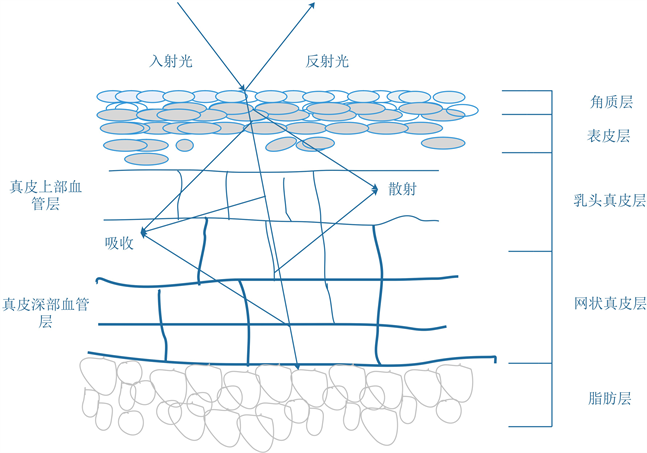Figure 1. The optical model of human skin

3. 皮肤组织对光的组要作用

3.1. 皮肤组织的吸收作用

${\mu }_{a-base}=7.84\ast {10}^{8}\ast {\lambda }^{-3.255}$ (1)

${\mu }_{a-mel}=6.6\ast {10}^{11}\ast {\lambda }^{-3.33}$ (2)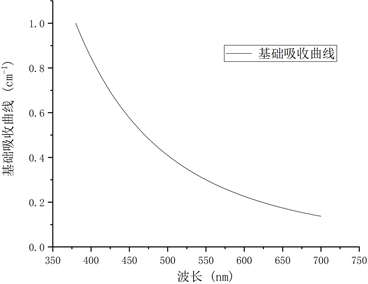Figure 2. Curves of melanin and basal absorption

${\mu }_{a-epi}=\left(1-{f}_{mel}\right){\mu }_{a-base}+{f}_{mel}\ast {\mu }_{a-mel}$ (3)Table 1. Dermal data and blood ratio

${\mu }_{a-ohb}=\frac{{\epsilon }_{ohb\left(\lambda \right)}}{66500}{c}_{hb}\ast \gamma$ (4)

${\mu }_{a-dhb}=\frac{{\epsilon }_{dhb\left(\lambda \right)}}{66500}{c}_{hb}\ast \left(1-\gamma \right)$ (5)

${\mu }_{a-bil}=\frac{{\epsilon }_{bil\left(\lambda \right)}}{585}{c}_{bil}$ (6)

${\mu }_{a-der}={f}_{blood}\ast \left({\mu }_{H}+{\mu }_{a-bil}\right)+\left(1-{f}_{blood}\right)\ast {\mu }_{a-base}$ (7)

$\begin{array}{l}{f}_{blood}={V}_{blood}/{V}_{der}=S\ast {h}_{blood}/S\ast {h}_{der}\\ =\left(S\ast 150\ast 0.04+S\ast 80\ast 0.3+S\ast 1500\ast 0.04+S\ast 100\ast 0.1\right)/S\ast \left(150+80+1500+100\right)\\ =0.0546\end{array}$ (8)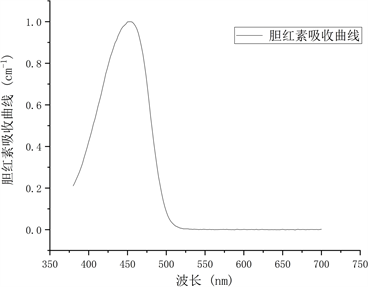Figure 3. Absorption curves of hemoglobin and bilirubin

3.2. 皮肤组织的散射作用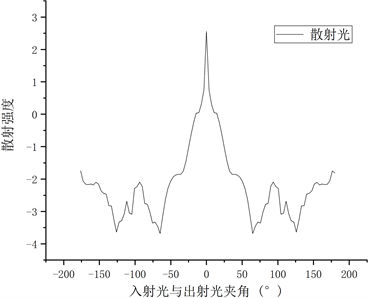(a)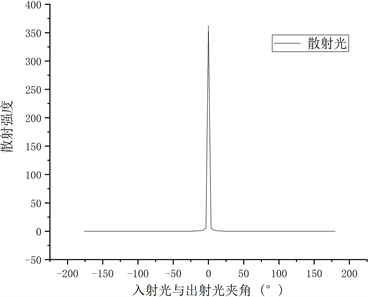(b)

Figure 4. (a) Angle between entrance and exit rays; (b) The logarithm of data (a)

${\mu }_{s}={\mu }_{s-epi}+{\mu }_{s-der}$ (9)

${\mu }_{s-epi}\approx {\mu }_{s-der}$ (10)

${\mu }_{s-mie}=2\ast {10}^{5}\ast {\lambda }^{-1.5}$ (11)

${\mu }_{s-Rayleigh}=2\ast {10}^{12}\ast {\lambda }^{-4}$ (12)

${\mu }_{s}={\mu }_{s-epi}+{\mu }_{s-der}$ (13)

4. 计算与分析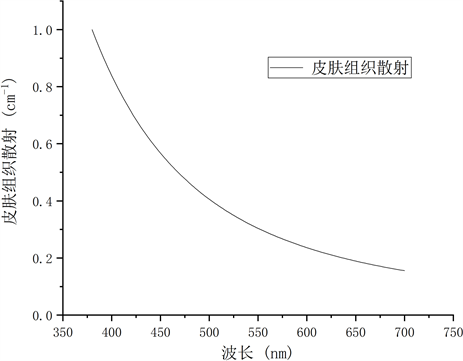Figure 5. Scattering curves of skin tissues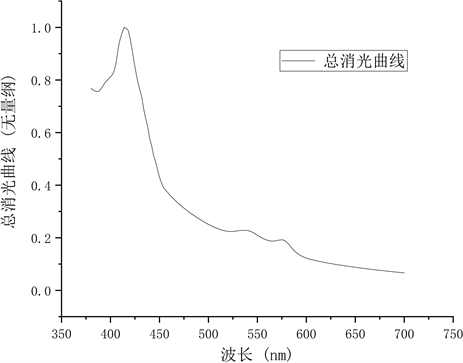Figure 6. Total extinction curve of skin

5. 结语

NOTES

*通讯作者。

  Lisenko, S.A. and Kugeiko, M.M. (2014) Method for Estimating Bilirubin Isomerization Efficiency in Phototherapy to Treat Neonatal Jaundice. Journal of Applied Spectroscopy, 81, 834-842. https://doi.org/10.1007/s10812-014-0013-8  杜立中. 新生儿高胆红素血症 [M]. 北京: 人民卫生出版社, 2015.  Stokowski, L.A. (2006) Fundamentals of Phototherapy for Neonatal Jaundice. Advances in Neonatal Care Official Journal of the National Association of Neonatal Nurses, 6, 303-312. https://doi.org/10.1016/j.adnc.2006.08.004  Bhutani, V.K., Fetusnewborn, C.O. and Pediatrics, A.A.O. (2011) Phototherapy to Prevent Severe Neonatal Hyperbilirubinemia in the Newborn Infant 35 or More Weeks of Gestation. Pediatrics, 128, 1046-1052. https://doi.org/10.1542/peds.2011-1494  Ebbesen, F., Madsen, P., St Vring, S., et al. (2007) Therapeutic Effect of Tur-quoise versus Blue Light with Equal Irradiance in Preterm Infants with Jaundice. Acta Paediatrica, 96, 837. https://doi.org/10.1111/j.1651-2227.2007.00261.x  杨光英, 吴晓翠. 新生儿黄疸治疗进展[J]. 临床儿科杂志, 2003, 21(4): 249-250.  Donner, C., Weyrich, T., D’Eon, E., et al. (2008) A Layered, Heterogeneous Reflectance Model for Acquiring and Rendering Human Skin. ACM Transactions on Graphics, 27, 1-12. https://doi.org/10.1145/1409060.1409093  Krishnaswamy, A. and Baranoski, G.V.G. (2004) A Study on Skin Optics. Technical Report CS-2004-01, 1-17.  陈荣, 曾海山, 谢树森, 等. 皮肤的光学模型[J]. 激光生物学报, 2005, 14(6): 401-404.  Angelopoulou, E. (2001) Understanding the Color of Human Skin. Proceedings of SPIE-The International Society for Optical Engineering, 4299, 243-251.  Meglinski, I.V. and Matcher, S.J. (2002) Quantitative Assessment of Skin Layers Absorption and Skin Reflectance Spectra Simulation in the Visible and Near-Infrared Spectral Regions. Physiological Measurement, 23, 741. https://doi.org/10.1088/0967-3334/23/4/312  Jacques, S.L. (1998) Skin Optics Summary. Oregon Medical Laser Center News. http://omlc.org/news/jan98/skinoptics.html  Prahl, S. (1999) Optical Absorption of Hemoglobin. http://omlcorg/spectra/hemoglobin/indexhtml  甘汝婷, 郭震宁. 新生儿黄疸治疗仪用LED光源光谱功率分布匹配[J]. 中国光学, 2014, 7(5): 794-800.  Lee, R., Mathewsroth, M.M., Pathak, M.A., et al. (1975) The Detection of Carotenoid Pigments in Human Skin. Journal of Investigative Dermatology, 64, 175-177. https://doi.org/10.1111/1523-1747.ep12533341  郑国铝. 细胞生物学[M]. 北京: 高等教育出版社, 1992.  叶玉堂, 肖峻, 饶建珍. 光学教程[M]. 北京: 清华大学出版社, 2011.  Georgios, N.S.P.D., Janeta, N.P.D., Michael, A.L.M.S., et al. (2010) Infant Skin Microstructure Assessed in Vivo Differs from Adult Skin in Organization and at the Cellular Level. Pediatric Dermatology, 27, 125. https://doi.org/10.1111/j.1525-1470.2009.00973.x  Walsh, R.J. (1963) Variation in the Melanin Content of the Skin of New Guinea Natives at Different Ages. The Journal of Investigative Dermatology, 42, 261-265. https://doi.org/10.1038/jid.1964.59